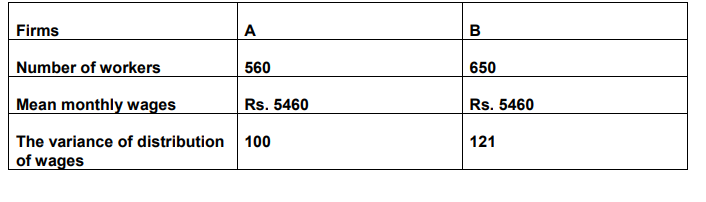Deepak Scored 45->99%ile with Bounce Back Crack Course. You can do it too!

# The following results show the number of workers and the wages paid to them in two factories A and B of the same industry.

Question:

The following results show the number of workers and the wages paid to them in two factories A and B of the same industry.(i) Which firm pays a larger amount as monthly wages?

(ii) Which firm shows greater variability in individual wages?

Solution:

(i) Both the factories pay the same mean monthly wages.

For factory A there are 560 workers. And for factory B there are 650 workers.

So, factory A totally pays as monthly wage $=(5460 \times 560)$ Rs. $=3057600 \mathrm{Rs}$.

Factory B totally pays as monthly wage $=(5460 \times 650)$ Rs. $=3549000 \mathrm{Rs}$.

That means, factory B pays a larger amount as monthly wages.

(ii) Mean wages of both the factories are the same, i.e., Rs. 5460 .

To compare variation, we need to find out the coefficient of variation (CV).

We know, CV = $\frac{\mathrm{SD}}{\text { Mean }} \times 100$ where SD is the standard deviation.

The variance of factory $A$ is 100 and the variance of factory $B$ is 121 .

Now, SD of factory A =

$\sqrt{100}=10$

And, SD of factory B =

$\sqrt{121}=11$

Therefore,

The $C V$ of factory $A=$

$\frac{10}{5460} \times 100=.183$

The $\mathrm{CV}$ of factory $\mathrm{B}=$

$\frac{11}{5460} \times 100=.201$

Here, the $C V$ of factory $B$ is greater than the $C V$ of factory $A$.

Hence, factory B shows greater variability.